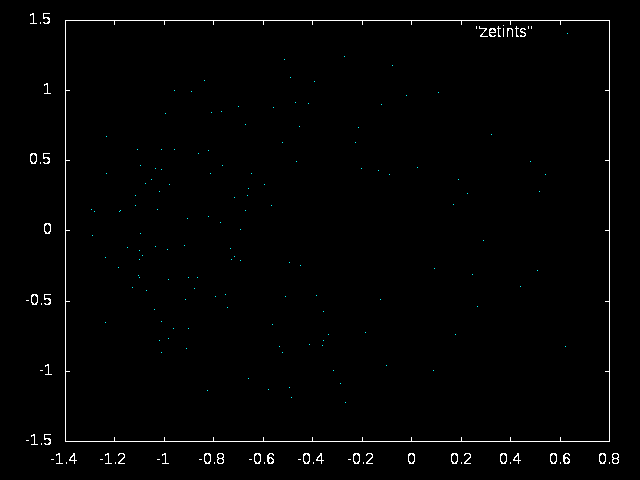lattice of (topological) pants(just imagine this extends to infinity…)
probably has some meaning in Teichmuller theory, but I’m a complex analyst, and want to string lacunaries across those circles, and insist that we can only link the pants when the lacunaries match.

an integral related to the zeta function

The integral in question is:$\int_{1/2}^{\infty} \left[\zeta\left(t+i\mathrm{Im}(\rho_{n})\right)-1\right] dt$

Since the limit starting from a nontrivial root going parallel to the real axis of the zeta function is 1, we may subtract that off so that it converges.

144 evaluations, text file, done with mpmath

What’s amazing, is that when the values of this integral corresponding to nontrivial roots of the zeta function are plotted, some interesting structure revealed: